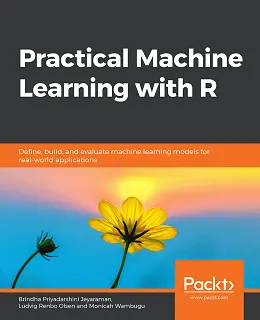# Practical Machine Learning with R### eBook Details:

• Paperback: 416 pages
• Publisher: WOW! eBook (August 30, 2019)
• Language: English
• ISBN-10: 1838550135
• ISBN-13: 978-1838550134

### eBook Description:

Practical Machine Learning with R: Understand how machine learning works and get hands-on experience in using R to build algorithms that can solve several real-world problems

With huge amounts of data being generated every moment, businesses need applications that apply complex mathematical calculations to data repeatedly and at speed. With machine learning techniques and R, you can easily develop these kinds of applications in an efficient way.

Practical Machine Learning with R begins by helping you grasp the basics of machine learning methods, while also highlighting how and why they work. You will understand how to get these algorithms to work in practice, rather than focusing on mathematical derivations. As you progress from one chapter to another, you will gain hands-on experience of building a machine learning solution in R. Next, using R packages such as rpart, random forest, and multiple imputation by chained equations (MICE), you will learn to implement algorithms including neural net classifier, decision trees, and linear and non-linear regression. As you progress through the book, you’ll delve into various machine learning techniques for both supervised and unsupervised learning approaches. In addition to this, you’ll gain insights into partitioning the datasets and mechanisms to evaluate the results from each model and be able to compare them.

• Define a problem that can be solved by training a machine learning model
• Obtain, verify and clean data before transforming it into the correct format for use
• Perform exploratory analysis and extract features from data
• Build models for neural net, linear and non-linear regression, classification, and clustering
• Evaluate the performance of a model with the right metrics
• Implement a classification problem using the neural net package
• Employ a decision tree using the random forest library

By the end of this Practical Machine Learning with R book, you will have gained expertise in solving your business problems, starting by forming a good problem statement, selecting the most appropriate model to solve your problem, and then ensuring that you do not overtrain it.